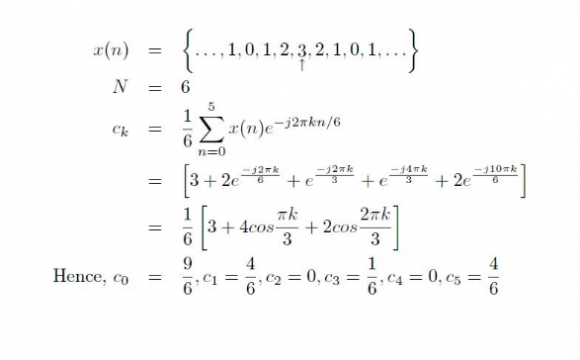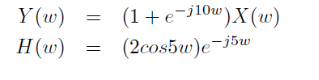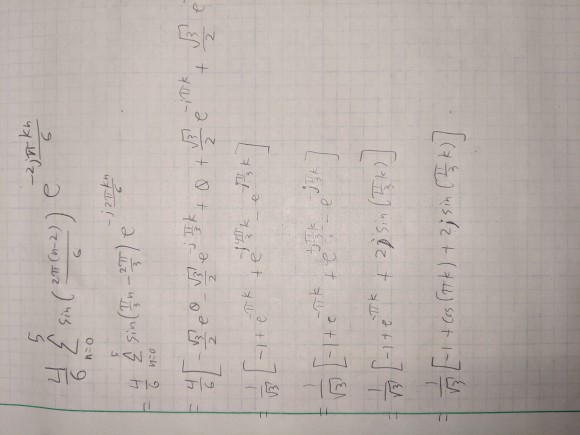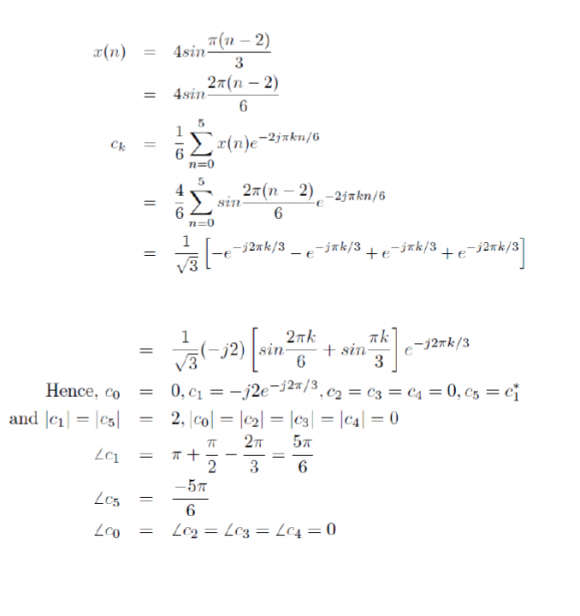## Simplifications With Eulers Equation (DTFT, DTFS)Started by 4 years ago14 replieslatest reply 4 years ago391 views

Hello,

I am new to DSP and am taking a DSP class in university right now. One item that I am having trouble understanding (and my textbook doesn't seem to be addressing) is how to simplify complex exponentials. I am aware of Euler's equation, however I think I am missing some tricks in how to solve these. Does anyone have a reference table that has a list of common simplifications using Eulers equation (or any DSP reference tables for that matter)?

Below are some specific instances that I don't quite understand:My question for the above is, how do we get the 4 cos(pi k /3) term and the 2 cos(2 pi k /3) term?

I understand that there is a relationship between cos(x) = .5*( e^i x + e^-x) but that doesn't seem to apply in the above? Unless if the 2 e^(-i 10 pi k /6 ) term is  equal to a 2 e^(+i 2 pi k/6) term (if so, then why?)

Another question pertains to the below image:

I understand how to setup this equation (for calculating DTFS coefficients). It is not clear to me how the book is able to get this result from the series. My guess is that because the sine term is delayed by (n-2) that somehow we can pull a complex exponential out of this equation. If so how can we do that?

Another question (sorry, I know I'm full of them today :p )I understand that H(w) = Y(w)/X(w), however how do we go from 1+e^(-j 10w) to 2 cos(5 w) * e^(-j 5w)

I cannot find any look up tables or references that show how to do this (if you have some please share).

Similar, how do we do the following:How can we pull the sin term out of the above complex exp. Whenever I calculate this I get

.5 - .5 * cos(2 w) + .5 * i * sin(2 w)

Any help or guidance on how to approach the above problems would be greatly appreciated.

Thank you so much :)

[ - ]Hi there,

you have to find a suitable factor so that you can always use the Euler formula, e.g. here:

[ - ]Hi,

Thanks again :)

[ - ]I send you the file Euler_1.png

[ - ]Thank you. Wow that makes so much more sense now. Thank you so much for answering  that question :)

While I have you, can you help shed some light on the below as well?I'm not sure how they get the below result from the above calculation. I believe that they are somehow using a property of the sin() to extract a complex exponential. Any thoughts?

Thanks again.

[ - ]Upon a reread, it isn't even that hard:

$$\sin( \frac{2}{3}\pi ) = \frac{\sqrt{3}}{2}$$

Similar for the other terms.

Sorry, I should have looked closer first.

+++++++++++++++++++++

The trick here is

$$i = e^{i\frac{\pi}{2}}$$

combined with:

$$\sin( \theta ) = \frac{e^{i \theta} - e^{-i \theta}}{2i}$$

Again, you should read my article for a intuitive understanding of these equations.

I would also recommend: Angle Addition Formulas from Euler's Formula

[ - ]Thank you. I will read those articles. Thanks again. I greatly apprecate it.

[ - ]So I worked out the problem using some of the tricks that you shared and this is what I got ( I did read over your blogs, they were helpful :)This answer is not adding up with the books answer. Do you see any mistakes that I am making?

Thanks again.

[ - ]That's hard for me to read.  Since you didn't post the book's answer, I can't tell you whether it's equivalent.  The thing with trig expression is they are incredibly transformable into equivalent expressions using trig identities.  You could also work this problem the hard way, using my original hints, to reduce it to a summation equation of exponentials and then apply the geometric summation formula.  It would be a good exercise.  Just compare your answer to the books.  Look at the parts that match and those than don't.  Trace the mismatch back in your logic to see if you can find an error.  It is very easy to drop a sign, or forget an "i".  The structure of your answer looks correct.
[ - ]Sorry, it might be helpful if I include the book answers :p

But ya, as best as I can tell, I don't think I am dropping any signs or missing any 'i' complex values.

Also, I agree with you that there might be many different forms of the final answer, but regardless of the form, they should all have the same value for specific values of k (k=0,1,2,3,4,5). Whenever I evaluate my answer for the various values of k, I don't get the same values for ck (again for k=0,1,2,3,4,5). This makes me think that I did something wrong.[ - ]I highly recommend that you read my blog The Exponential Nature of the Complex Unit Circle

$$e^{i \theta} = \cos( \theta ) + i \sin( \theta )$$

$$e^{-i \theta} = \cos( -\theta ) + i \sin( -\theta ) = \cos( \theta ) - i \sin( \theta )$$

$$e^{i \theta} + e^{-i \theta} = 2 \cos( \theta )$$

$$\cos( \theta ) = \frac{e^{i \theta} + e^{-i \theta}}{2}$$

So, on your second question:  (Using a math "i" instead of an engineering "j")

$$\left( 1 + e^{-i10w} \right) = e^{-i5w} \left( e^{i5w} + e^{-i5w} \right) = e^{-i5w} \cdot 2 \cos(5w)$$

The examples from your first part should now be clearer.

On your last part, the corresponding equation is:

$$e^{i \theta} - e^{-i \theta} = 2i \sin( \theta )$$

[ - ]Thank you for the clarification. I will most certainly read your blog.

:)

[ - ][ - ]